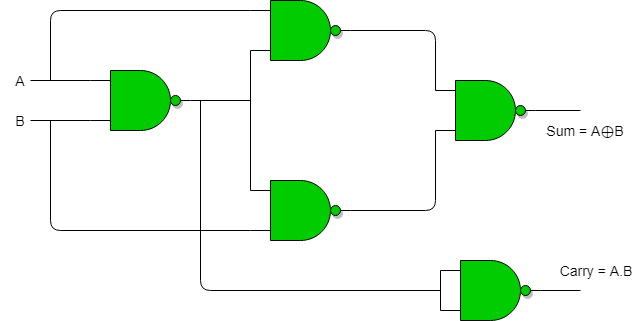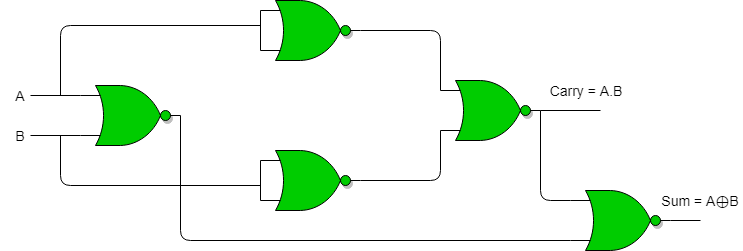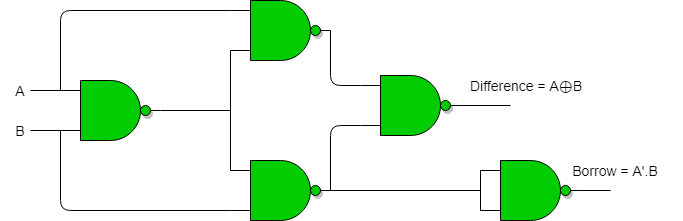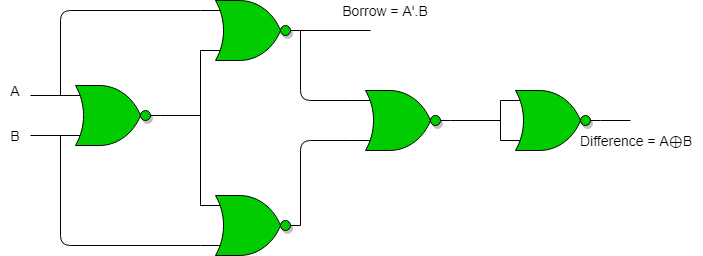# Half Adder and Half Subtractor using NAND NOR gates

• Difficulty Level : Medium
• Last Updated : 22 Feb, 2019

Implementation of Half Adder using NAND gates :
Total 5 NAND gates are required to implement half adder.Implementation of Half Adder using NOR gates :
Total 5 NOR gates are required to implement half adder.Implementation of Half Subtractor using NAND gates :
Total 5 NAND gates are required to implement half subtractor.Implementation of Half Subtractor using NOR gates :
Total 5 NOR gates are required to implement half subtractor.